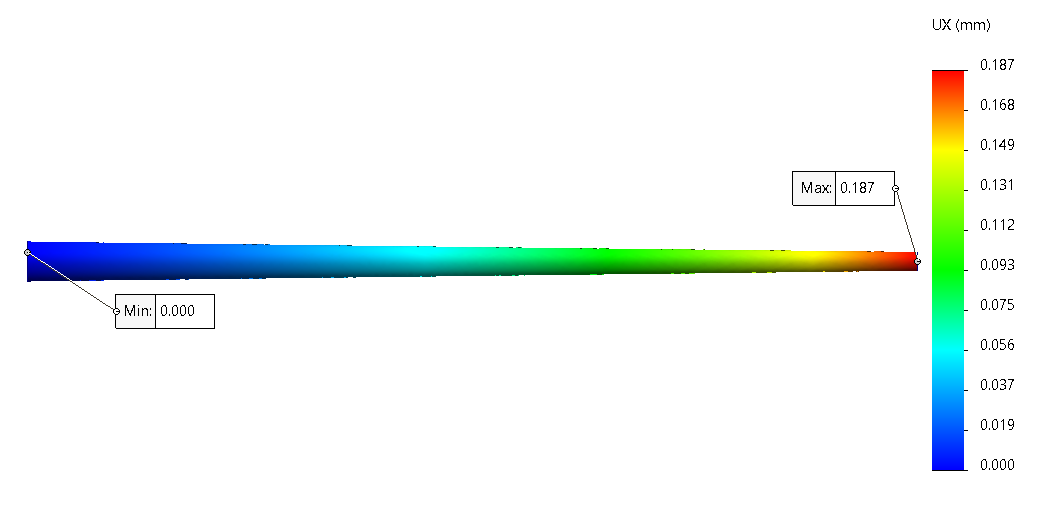## Enhancing Theoretical calculation with Virtual Validation using SOLIDWORKS Simulation

A steel rod circular in section, tapers from 3 cm diameter to 1.5 cm diameter in a length of 60 cm.Find how much its length will increase under a tensile force 22 kN. Take E = 2 x 105 N/ mm2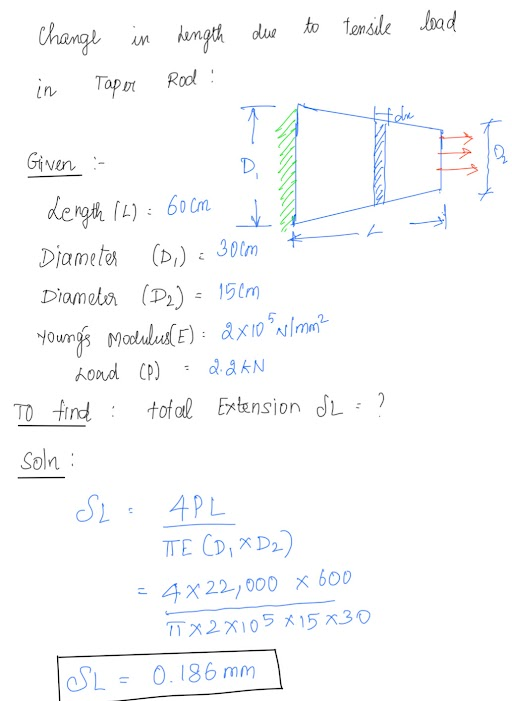The Hand calculated taper Rod deflection is 0.186 mm. We will follow the solution using Simulation Package. The Material Properties introduced in the software.

Create a Geometry to build in the software for given Dimensions: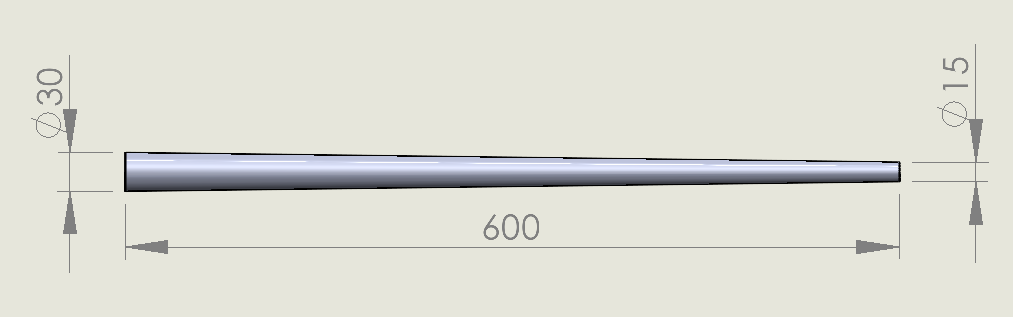Fig (1) 3D model of Taper Rod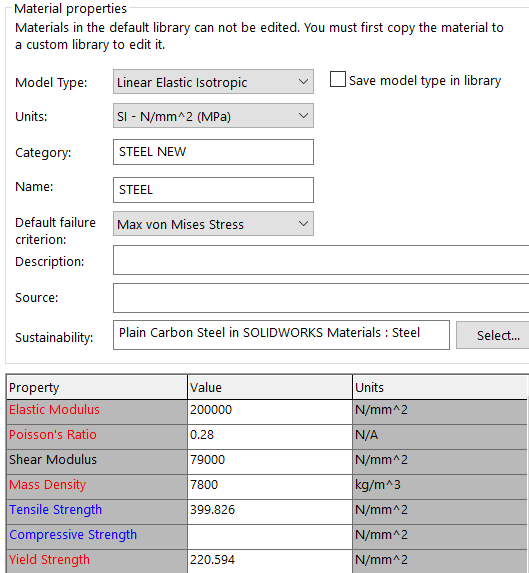Fig (2) Material Properties involved in the calculation

Boundary conditions involved as per theoretical conditions involved in the same.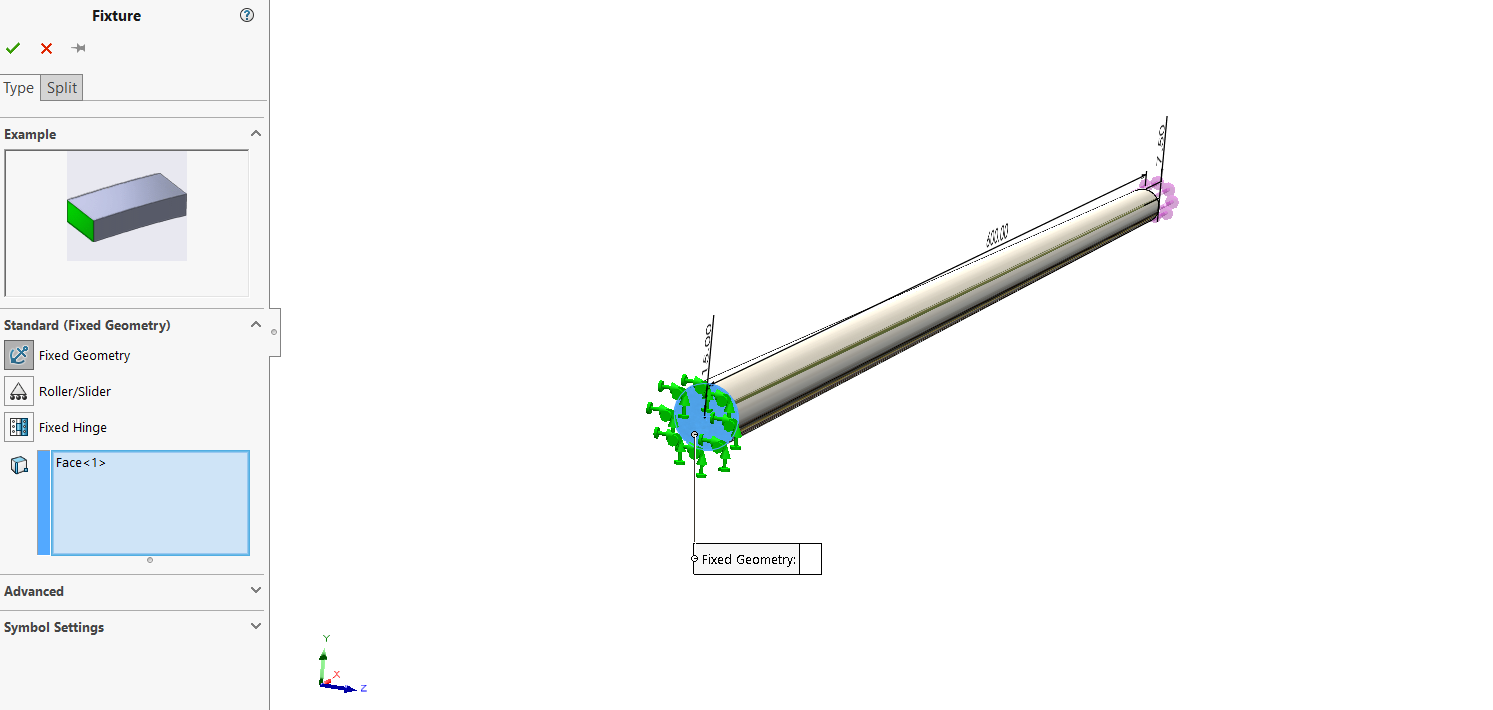Fig (3) Fixing at the larger end

For solid we can arrest the three Translation x, y, z and rotation of Three components will be eliminated.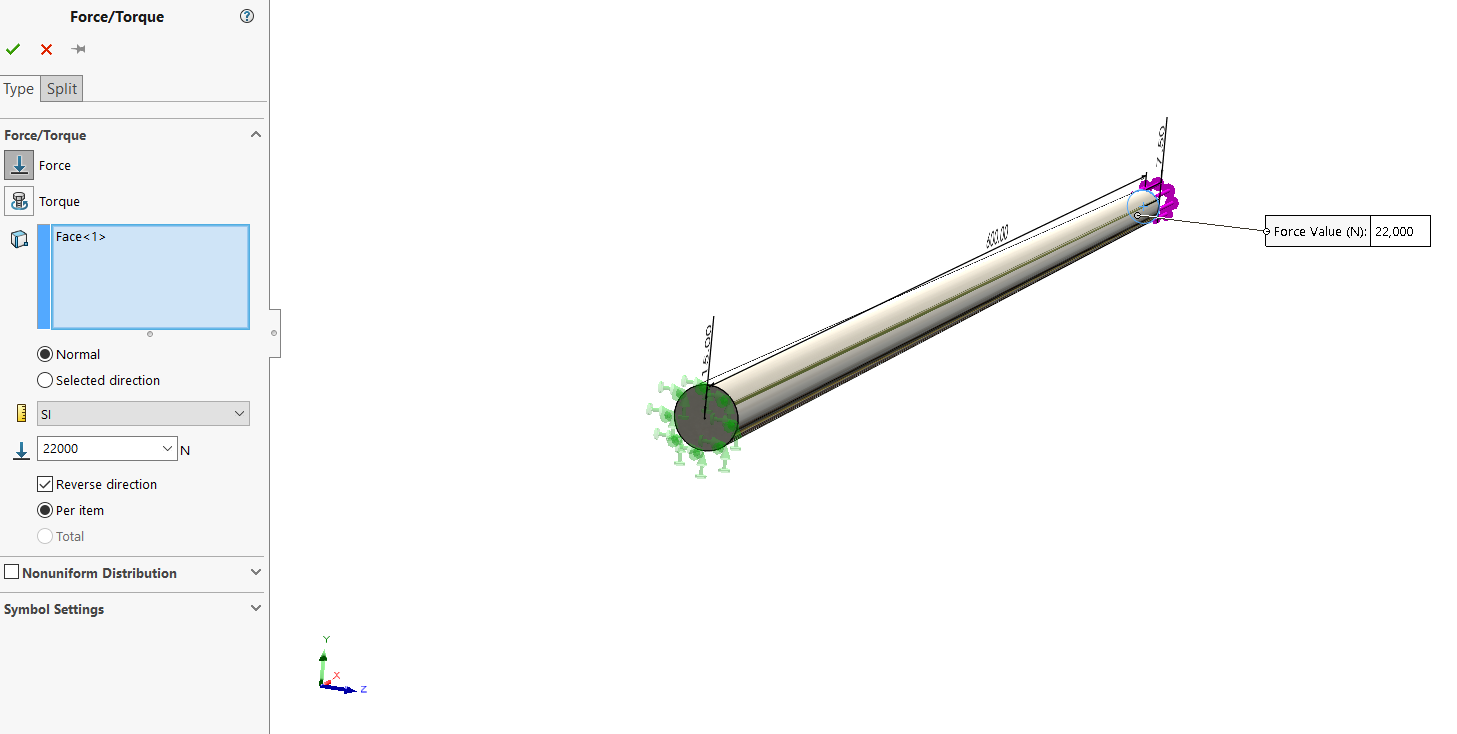Fig (4) Fixing at the smaller end